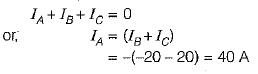Courses

# Test: Poly Phase AC Circuit

## 10 Questions MCQ Test Topicwise Question Bank for Electronics Engineering | Test: Poly Phase AC Circuit

Description
This mock test of Test: Poly Phase AC Circuit for Electrical Engineering (EE) helps you for every Electrical Engineering (EE) entrance exam. This contains 10 Multiple Choice Questions for Electrical Engineering (EE) Test: Poly Phase AC Circuit (mcq) to study with solutions a complete question bank. The solved questions answers in this Test: Poly Phase AC Circuit quiz give you a good mix of easy questions and tough questions. Electrical Engineering (EE) students definitely take this Test: Poly Phase AC Circuit exercise for a better result in the exam. You can find other Test: Poly Phase AC Circuit extra questions, long questions & short questions for Electrical Engineering (EE) on EduRev as well by searching above.
QUESTION: 1

Solution:
QUESTION: 2

### In a balanced three-phase star connected system, the phase voltages

Solution: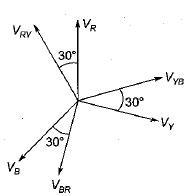Here, line-to-line voltage leads the respective phase voltage by 30°.

QUESTION: 3

### Three identical resistances are connected in delta against a balanced three-phase voltage supply. If one of the resistances be removed, the reduction in the power will be

Solution:

When all the resistance are present,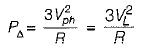(Since Vph= VL for Δ connection)
When one of the phase resistance is removed,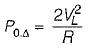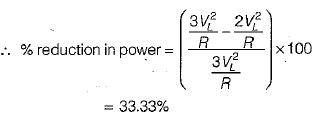QUESTION: 4

In a delta connected system, the line current are

Solution: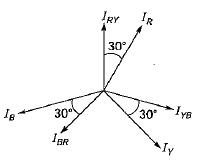QUESTION: 5

A balanced Y-connected load is supplied from a balanced 3-phase 400 V system. If the current in each phase is 15 A and lags the phase voltage by 30°, the total power will be

Solution: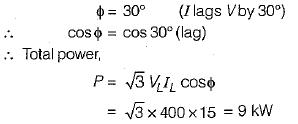QUESTION: 6

In a balanced three-phase circuit VAN= 1500 ∠20° V and VCN = 1500 ∠-100° V.

The value of VBN will be

Solution:

Since VCN lags VAN by 120°, therefore phase sequence will be ACB.
Here, VBN will lead VAN  by 120° but, will have the same magnitude.

so,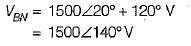QUESTION: 7

The value of the rms line voltage VL for the below circuit if the rms line voltage is 100 V will be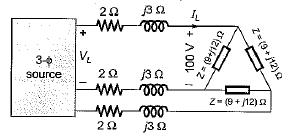Solution:

Converting the Δ-connected load in star equivalent, we have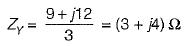So, line to neutral voltage for equivalent Y-load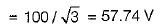∴ Line current,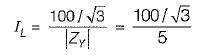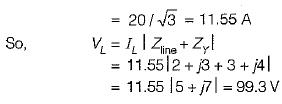∴ Line voltage at source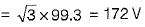QUESTION: 8

Two wattmeters, both have reading of 3 kW when connected for the two-wattmeter method with current coils in lines A and B of a 600 V ABC circuit having a balanced A load. The Δ phase impedance will be

Solution:

we have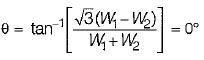(Since W1 = W2 = 3 kW)
or, cosθ= 1

Hence, the load will be purely resistive.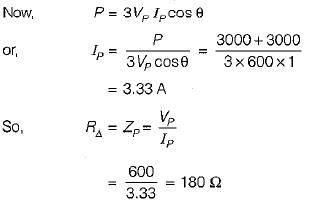QUESTION: 9

Three capacitances, each of C/3 Farads are connected in delta. Their equivalent star value for each capacitance is

Solution:

We know that,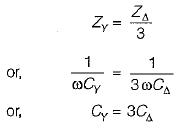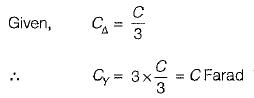QUESTION: 10

The instantaneous value of currents in both phases B and C of a 3-phase balanced system are -20 A. For a phase sequence of ABC, the instantaneous value of current in phase A is

Solution:

Since the system is balanced, therefore